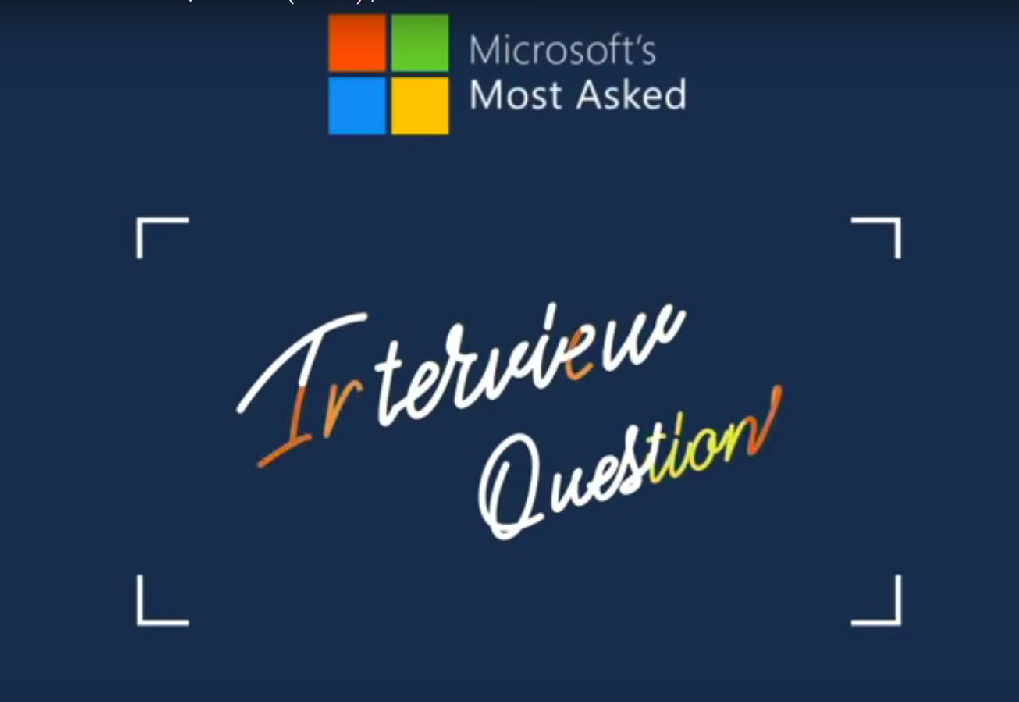Related Articles
• Difficulty Level : Medium
• Last Updated : 23 Apr, 2019

Like other product based companies, Microsoft also asks data structures and algorithms as part of their technical interview. Below is a of questions prepared from different Microsoft interview experiences.• Check if a Binary Tree is BST or not – Practice here
• Remove duplicates from a string, do it in-place – Practice here
• Given a rotated array which is sorted search for an element in it – Practice here
• Given two linked lists that represent two big numbers (numbers that can not be stored in an int or long long int), write a function that adds the numbers and store the result in a third list.
• Variations of above question like subtract two numbers or multiply two numbers represented by linked lists. – Practice here
• Print last 10 lines of a big file or big string.
• Clone a linked list with next and arbitrary (or random) pointer
• Connect nodes at same level. Practice here.
• Least common ancestor of a binary tree or a binary search tree
• Test case questions like test an ATM.
• Run length encoding. – Practice here
• Detect cycle in a linked list. Practice here.
• Given a sorted array of size n. Each element in array is unique and lies from 1 to n+1. Find the missing element. Write code and test cases for the same. – Practice here

• Check if a binary tree is balanced.
• Validate a given IP address
• Why do you want to join Microsoft?

• Two of the nodes of a BST are swapped. Correct the BST
• Draw a circle without floating point arithmetic